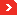Client Login# Volumetric Weight

### Airfreight

Length (cm) x Height (cm) x Width (cm) / 6000 = Volumetric Weight in KGS

### Seafreight

Length (m) x Height (m) x Width (m) = cubic metres. Seafreight has a 1:1 ratio ie 1000kgs = 1cbm
(except for US destination beyond Los Angeles).

### Courier

Length (cm) x Height (cm) x Width (cm) / 6000 = Volumetric Weight in KGS